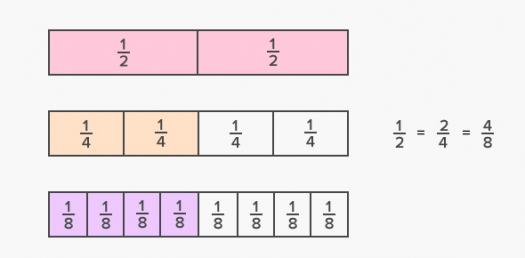# Eii Studyhelper Math Fraction

15 Questions | Total Attempts: 122Settings.

• 1.
What are similar fractions?
• 2.
Equivalent fractions are fractions that has the same value even they though they look different.  What word means the same with the underlined word?
• A.

Similar

• B.

Same numerator

• C.

Equal

• 3.
If the numerator is 4 and the denominator is 5, the fraction is 5/4.
• A.

True

• B.

False

• 4.
The sum of 2/5 and 4/5 is 7/5.
• A.

True

• B.

False

• 5.
The difference of 5/3 and 1/3 is 1/3.
• A.

True

• B.

False

• 6.
To give the fraction 1/8, the whole must be divided into 5.
• A.

True

• B.

False

• 7.
Two-thirds (2/3) is less than 1
• A.

True

• B.

False

• 8.
Five-sevenths (5/7) is less than 1.
• A.

True

• B.

False

• 9.
Five-fifths is equal to 1.
• A.

True

• B.

False

• 10.
If a cake was cut into 4 equal parts and Trina and her friends ate all.  What is the fraction Trina and her friends eaten?
• 11.
What is the sum 1/11 and 7/11?
• 12.
Valerie drove Makati to Alabang in 2/3 of an hour?  Which fraction is equivalent to 2/3?
• A.

4/6

• B.

6/6

• C.

3/6

• 13.
What is the missing fraction in 4/5 + ? = 9/5
• 14.
Rodrigo studied Math for 2/6 of an hour and Science for 3/6 of an hour.  How long did he study Math and Science?
• A.

1/6 of an hour

• B.

5/6 of an hour

• C.

6/6 of an hour

• 15.
4/7 and 5/7 are dissimilar fractions.
• A.

True

• B.

False

Related TopicsBack to top